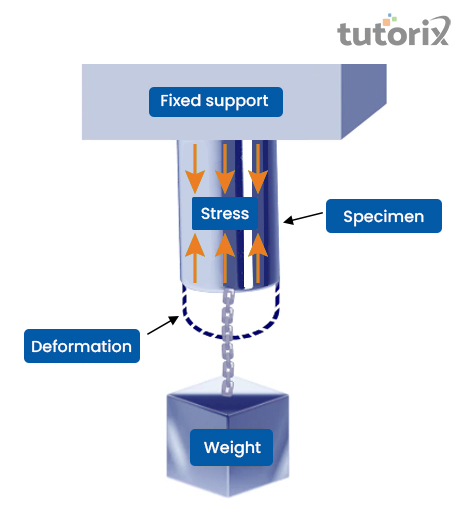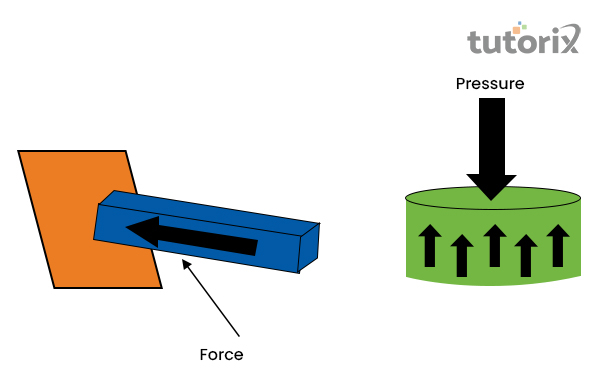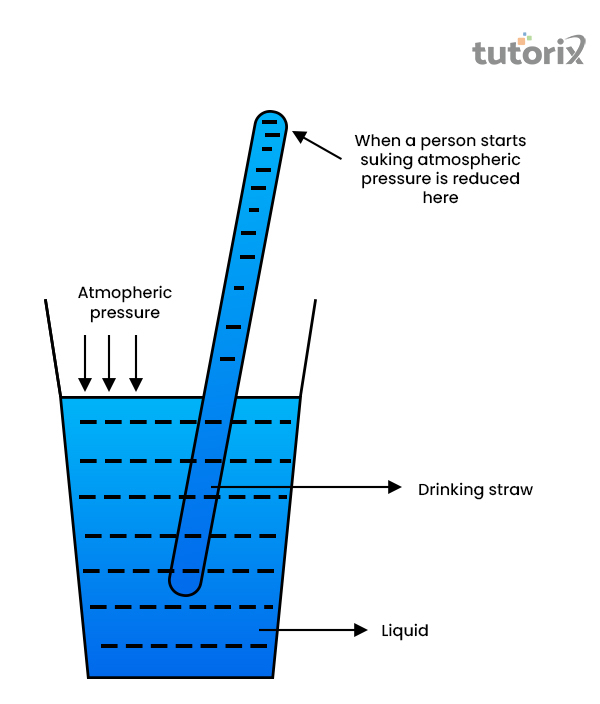# Difference Between Stress and Pressure

## Introduction

Stress and pressure are both forces that are often misinterpreted; however, pressure is defined as the physical force applied to an object. In simpler words, pressure is an amount of force that is applied per unit area. Pressure is majorly noticed as the unique property of thermodynamics and serves as a cause for the aspect of stress. On the other hand, stress is referred to as the material property and it occurs due to the result of the pressure exerted. However, both the forces are crucial as they are used in the field of physical science and engineering as it permits proper description and prediction of the behaviour of fluids, elastics and also of plastics.

## What is stress?

Stress in simpler terms is defined as the intensity that measures the force of internal resistance. However, this resistance force is generated at a particular point as a result of the application of pressure of force. The amount of internal force needs to apply per unit area (Han et al. 2019). This should be noted that the notion of stress is produced within the material body of the stated object. Therefore, the stress of a body can be expressed as, $\mathrm{\sigma\:=\:(-)\:F/A}$, where, $\mathrm{-F}$ is stated as the internal resistive force.Figure 1: Stress generated in specimen

### Application of stress

The notion of stress is majorly applied in the field of physical science and engineering and has several uses. The diagram noted for stress and strain determines a graphical display for measuring the strength as well as the elasticity of several materials.

However, shear stress is observed in solid and that results in several actions that include, twisting for a bar of mental, on a basis of an axis of longitude. This occurs when the event of tightening of a screw is done. The flow of liquids and gasses occurs with the help of shear stress in fluids (Whatispiping, 2022). In the passage of the aeroplane through the air also evidences the application of shear stress. For continuous deformation, shear stress is applied in true fluids.

## Definition of Pressure

The term pressure can be defined as the intensity with which the external forces act at a particular point. This is a number of forces applied per unit area that are external in nature. However, pressure seems to act like a normal to the surface of the object, it acts and this force is applied in both the forms of matters such as, liquids and gasses.

The pressure is represented as, p = F/A, where F is denoted as the positive directive force. The notion of pressure can be measured physically with the help of several instruments that include, a barometer, manometers, pressure gauges and many more.Figure 2: Force

### Pressure: Formula and application

The force that is projected on the basis of per unit area on an object is usually mentioned by the term pressure. For the representation of this term, Pascal is used as the SI unit. The definition of a pascal can be stated as the one-newton force that is applied over a surface area of one-meter square (Physicsgoeasy, 2022). Based on this, the formula of pressure has been denoted and the mathematical representation of this formula is Pressure = Force / Area.Figure 3: Application of air pressure in daily life

The application of pressure that can be seen in daily life is the atmospheric pressure. In a more simple diction, it can be stated that the air pressure is referred to here. In daily life, several activities have been performed by applying air pressure.

The body function of human beings daily survives the high pressure of the ai8r. On the other hand, while drinking with a straw, bullets fired from a gun are examples of the application of air pressure.

### Stress and Pressure: Differences

The differences between pressure and stress are as follows −

Stress can be defined as the internal resistance force of the object that is deformed per unit basis. In contradiction, pressure is defined by the amount of force applied per unit area of an object's surface. The stress can be both positive and negative whereas, the pressure can be only determined as a positive one. The calculation of stress can only be possible by calculation of measuring strain. On the other hand, pressure can be measured physically by using gauges, manometers (Sciencedirect, 2022).

Pressure is always compressive but the stress can be shear, compressive and tensile. In a different direction, the magnitude of the stress is different, but the pressure remains the same in all directions.

## Conclusion

The pressure and the stress, both concepts are exemplified in this present tutorial. In the definition of pressure, the physical force is generally termed as pressure whereas the stress is defined as the material property of an object. These differences in definitions define that these two terms of physics behold some differences. Focusing on this statement, the current tutorial has explained the differences between these two concepts. The core difference is that the stress can be applied to the solid object whereas the pressure can be applied to liquids, fluids and gases as well.

### FAQs

Q1. Is pressure always compressive in nature?

In accordance with the laws of physics, the pressure is always considered to be acted normal to the surface and its nature of it is always compressive. In order to prepare a complete description of pressure, only the magnitude is used.

Q2. In physics, is the pressure is same as stress?

In several cases, the stress is used as a synonym for pressure but these two concepts are different from each other, the core difference is that the force applied externally is pressure but the stress is considered to be found internally which influences the object to change size or shape.